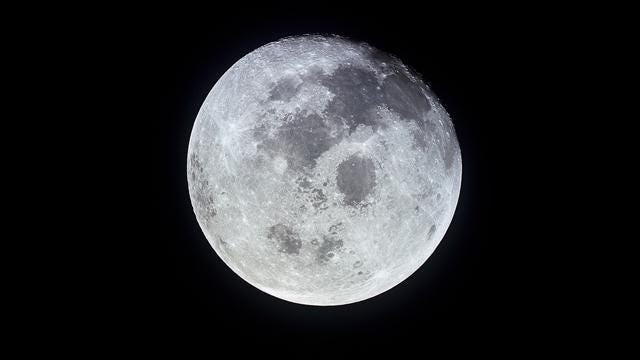Published on Jun 04, 2017

# Crazy Math: How to reach the Moon by folding a paper

Yes, you read it right. Folding a paper can get you to the Moon.Image Source- National Geographic

How many times can you fold a piece of paper. Assume we have a very fine paper which is only 0.001cm or 0.1mm thick. Let’s also assume the size of the paper is so big that you can fold it over and over as many times as you wish. How many times would you have to fold a piece of paper for it to be thick enough to reach the moon? Think for a moment.

Well, the answer may surprise you. You would need to fold the piece of paper 45 times for it to be thick enough to reach the moon.

Okay, So now let’s use some Math. Initially we have a paper which is 0.1mm thick. If we fold the paper once, it is now 0.2mm in thickness. If we fold it one more time, it is now 0.4mm thick. If we fold it one more time, it is now 0.8mm thick. Did you see the pattern? Every time we fold the paper it gets twice as thick. If we fold this paper 17 times we’ll get a thickness of 2 to the power of 17, which is 131cm and that equals just over 4 feet. If we were able to fold it 25 times, then we would get 2 to the power of 25, which is 33,554cm and that equals over 1,100 feet. It’s worthwhile to stop here and think for a moment. Folding a paper in half, even a paper so thin, 25 times would give us a paper almost a quarter of a mile. This type of growth is called Exponential Growth. If we fold a paper 30 times, the thickness reaches 6.67 miles which is about the average height that planes fly. 40 times, the thickness is nearly 7000 miles or the average GPS satellite’s orbit. 45 times, the thickness is now over 250,000 miles and the distance between earth and moon is around 239,000 miles. So we finally reached the moon. And by folding it one more time i.e. 46th time we can now come back to earth.

Thank you for your time. If you liked the post please hit the red heart button.

Follow the publication for more Crazy Math Facts.

Originally published on Medium By Priyansh Rastogi on June 4, 2017.